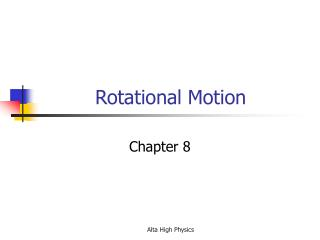Download PresentationRotational Motion

Loading in 2 Seconds...

# Rotational Motion - PowerPoint PPT Presentation

Rotational Motion. Chapter 8. Important Variable. θ (theta) – angular displacement – radians ω (omega) – angular velocity – radians/sec α (alpha) = angular acceleration – radians/sec 2 t – still time and still in seconds. r. L. θ. Measuring Angular Displacement. θ = L/rI am the owner, or an agent authorized to act on behalf of the owner, of the copyrighted work described.
Download Presentation## Rotational Motion

An Image/Link below is provided (as is) to download presentation

Download Policy: Content on the Website is provided to you AS IS for your information and personal use and may not be sold / licensed / shared on other websites without getting consent from its author.While downloading, if for some reason you are not able to download a presentation, the publisher may have deleted the file from their server.

- - - - - - - - - - - - - - - - - - - - - - - - - - E N D - - - - - - - - - - - - - - - - - - - - - - - - - -
Presentation Transcript
1. Rotational Motion Chapter 8 Alta High Physics

2. Important Variable • θ (theta) – angular displacement – radians • ω (omega) – angular velocity – radians/sec • α (alpha) = angular acceleration – radians/sec2 • t – still time and still in seconds Alta High Physics

3. r L θ Measuring Angular Displacement • θ = L/r • 360° = 2π radians = 1 revolution • v= ωr = Linear velocity = angular velocity x radius • a = αr Linear acceleration = angular acceleration x radius Alta High Physics

4. Sample Problems • If an exploding fireworks shell makes a 10° angle in the sky and you know it is 2000 meters above your head, how many meters wide is the arc of the explosion? Alta High Physics

5. Solution θ = L/r θ = 10° x π rad/180° = 0.17 rad 0.17 rad = L/2000 meters L = 2000 meters x 0.17 rad = 349 meters Alta High Physics

6. Sample Problems • Convert the following measures from Radians to degrees: • 3.14 rad • 150 rad • 24 rad Alta High Physics

7. Solution • 3.14 rad x 180°/π rad = 180° • 150 rad x 180°/π rad = 8594° • 24 rad x 180°/π rad = 1375° Alta High Physics

8. Sample Problems • What is the linear speed of a child seated 1.2 meters from the center of a merry-go-round if the ride makes one revolution in 4 seconds? • What is the child’s acceleration? Alta High Physics

9. Solution a) v = ωr and ω=2π rad/4 sec = 1.6 rad/s since r = 1.2 m then v = 1.58 rad/s x 1.2 m = 1.9 m/s b) Since the linear velocity is not changing there is no linear or tangential acceleration, but the child is moving in a circle so there is centripetal or radial acceleration which you will recall fits the equation: a c = v2/r and since v = ωr ac = ω2r so a c = (1.9 m/s)2/1.2 m = 3.0 m/s2 or ac = ω2r = (1.6 rad/s)2(1.2 m) = 3.0 m/s2 Alta High Physics

10. Angular Kinematic Equations LinearAngular V = v0+at ω=ω0+ αt x = v0t+½at2θ=ω0t+½ αt2 V2 = v02+2ax ω2=ω02+2αθ Vavg = (v0+vf)/2 ωavg=(ω0+ωf)/2 Alta High Physics

11. Sample Problem A angular velocity of wheel changes from 10 rad/s to 30 rad/s in 5 seconds. • What is its angular acceleration? • What is its angular displacement while it is accelerating? Alta High Physics

12. Solution α = (ωf – ω0)/t = =(30 rad/s – 10 rad/s)/5 sec = 4 rad/s2 θ = ω0t = ½αt2 = 10 rad/s + ½(4 rad/s2)(5 sec)2 = 100 rad Alta High Physics

13. Torque • Torque = Fr = force x radius • Torque is measured in newton•meters which means that it has the same units as work in a linear system. Alta High Physics

14. Rotational Inertia • The rotational inertia of an object depends upon its shape and its mass. • The equations for each shape are given on page 223 in the text • Torque = Iα = rotational inertia x angular acceleration • Hence Fr = Iα Alta High Physics

15. Sample Problem A force of 10 newtons is applied to the edge of a bicycle wheel (a thin ring mass 1 kg and radius of 0.5 meters). What is the resulting angular acceleration of the wheel? If the wheel was at rest when the force was applied and the force is applied for 0.4 seconds what is the angular velocity of the wheel immediately after it is applied? Alta High Physics

16. Solution Since Fr = Iαthen α = Fr/I and since the wheel is a thin ring: I = mr2 = (1 kg)(0.5 m)2 = 0.25 kg m2 So α = (10 N)(0.5 m)/0.25 kg m2 = 20 rad/s2 ωf = ω0 + αt = 0 rad/s + (20 rad/s2)(0.4 sec) = 8 rad/s Alta High Physics

17. Problems Types • Finding angles when distance and length of arc are known • Converting from revolutions to radians and radians to degrees • Finding angular velocity and angular acceleration if radius and their linear counterparts are known • Using angular kinematic equations • Calculating Torque Alta High Physics